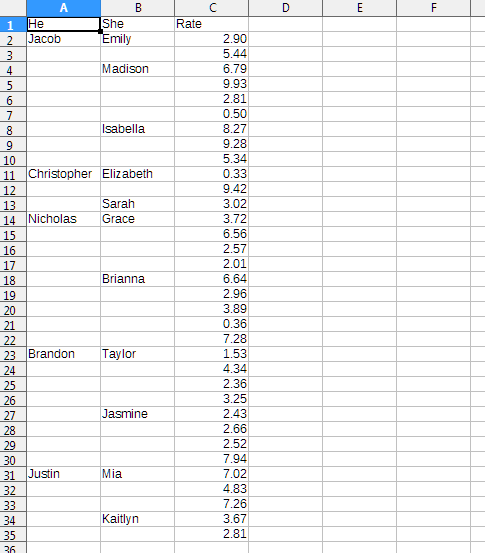# [SOLVED] Fill blank cells with value from above

Does Calc has such special option as there is in Excel (Go to special)?

I want to do exactly the same thing as on this tutorial:

Fill Blank Cells in Excel With Value from Above

Or maybe there is a function for this?

You can done this with simple formula and Ctrl+Shift+V (Paste Special - Skip Empty Cells)2 Likes

like that ideaGreat. It works. One more question. It looks like you automatically filled column D and E with function. What was it? A shortcut or just a video is cut?

It’s simple. Please note that in the video, the mouse cursor becomes a “cross”. This happens when you hover over the lower-right corner of the selected cell (bold square dot). Double-clicking in this position works like “fill down to the end of the range”. “End of range” is the end of a column of cells without gaps in the adjacent column (in the video it is column C)

Thank you very much.

And about your question. Yes, you can highlight blank cells in a range. This is very easy to do with a find regular expression `^\$` (start of text - nothing - end of text). However, having received several selected ranges of empty cells, you will not be able to enter a formula in them in one motion. That is why I suggested using the formula in a free auxiliary range.

Thank you. I applied your method and got the desired results. However, I could not understand the logic behind filling those columns with zeroes first, before using paste special option. Could you kindly explain?

The formula in cell D2 looks like `=D1`. At the time of writing the formula, there is nothing in cell D1, so the result of the formula is 0 (this is the usual behavior of Calc - so it lets you know that cell D2 is not empty). The same thing happens in cell E2. After multiplying the formulas down, each cell contains the “value” (actually a reference) of the cell one row upper. When inserting values from columns A:B, some of the formulas are replaced with non-empty values, and the formulas begin to display these values.

1 Like